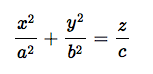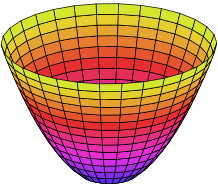# Paraboloid: Definition

A paraboloid is a quadric surface that has no center of symmetry but has one symmetry.

One type is the surface of revolution we get when we rotate a parabola around its center axis, but that’s not the only type there is. Some look more like saddles. Every member of this family of quadric surfaces, though, can be generated by a moving parabola directed by another parabola.

## Types of Paraboloid

There are two types of paraboloid:

1. Elliptic,
2. Hyperbolic.

## 1. Elliptic Paraboloid

The mini video shows the different shapes of an elliptic paraboloid:

These can all be described by the equation:• The vertical cross sections are parabolas, which all open in the same direction; The sign of c tells us whether they open upwards or downward: negative (down); positive (up).
• The horizontal cross sections are circles, if a and b are equal. If a≠b, the cross sections are oval.The simplest elliptic paraboloid has the equation z = x2 + y2.

## 2. Hyperbolic Paraboloid

A hyperbolic paraboloid differs from an elliptic in that it opens up in more than one direction. It can be designated by the equationThe hyperbolic form is shaped like a saddle, but again the sign of c tells us whether it opens upwards or downwards.## Applications of Paraboloids

Circular paraboloids are often used in mirrors and antennas, such as the satellite antennas you often see outside of buildings. This is because each of those cup-shaped surfaces has a focal point on an axis which reflects light into a parallel beam, parallel to the axis. Waves of light or other energy that hit the insides of the paraboloid will also be concentrated at the focal point.

Hyperbolic paraboloids are called double-ruled surfaces because they have two families of mutually skew lines. They are often used in architecture since they are creating a strong structure and straight iron beams can be used in their construction. The trademark shape of Pringles is also the shape of a truncated hyperbolic.

## References

Dunfield, N. Elliptic Paraboloid. Retrieved September 20, 2019 from: https://faculty.math.illinois.edu/~nmd/quadrics/ellparab.html

CITE THIS AS:
Stephanie Glen. "Paraboloid: Definition" From StatisticsHowTo.com: Elementary Statistics for the rest of us! https://www.statisticshowto.com/paraboloid/